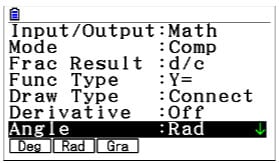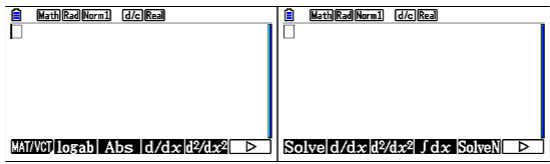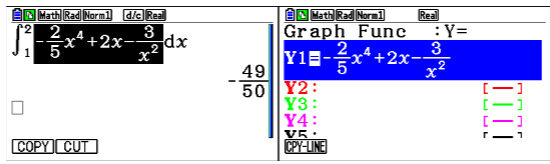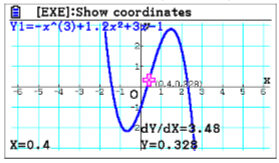Alleviate exam time pressure by teaching efficient use of the fx-CG50 - Casio Calculators

# Alleviate exam time pressure by teaching efficient use of the fx-CG50

Feb 2023 Longer Read: 6 MinExam season will soon be here. We’ve all seen students struggling
with pre-exam stresses, and while, as teachers, we can’t take the exam for them, we can
help them make the most of the time they have in the exam hall. In Maths exams, getting
students to use their calculator efficiently helps them make the most of their time and can
alleviate some of those exam pressures.

We asked Mathematician Simon May to share some practical features on the fx-CG50, which are helpful for students preparing for their and during Maths exams.

## Setup – the best place to start

“Any calculator can be overwhelming if not used right,” Simon explains. “The best place we
recommend starting is the setup because it allows students to find a setup they like.”
For example, by setting the Input/Output to “Math”, students can make sure they can input
and get output in the same natural format as seen in the question without any special
calculator syntax, which would be very helpful. You can easily set the mode of calculation,
how to display fractions, function type etc. It is useful for the students to turn on the
derivative from here as it would help them when doing certain calculations.
Students should pay close attention to the “Angle” field. Radian and Degree are the two
most widely used units of measuring angles. In most A-level exams, the calculations are
done using Radians. But sometimes, the examiners can ask for calculations using Degrees
to trick the students.There is also a field to change the “Complex Mode”, allowing Further Maths students to
Another interesting setting here is the “Display” field, and usually, the “Norm1” option is best
suited for it because it answers with full decimal places. It is wise to remember that students
can lose marks if they use a rounded-off answer in further calculations.
When highlighting each field using the scroll pad, the available options are displayed at the
bottom of the screen. The students can select which option they need for each field using
the function buttons F1 to F6.

## Exam mode – a student’s friend for an exam

“In an exam, since every second counts and students should not get accused of cheating,
it’s important for students to have only what’s required with them, let it be their pens, pencils
and water bottle,” Simon says. “It’s no exception for the calculator; only the needed settings
should be available, which also saves time. Enabling the exam mode is essentially the same
as resetting the main memory of the calculator, giving the students a new, clean slate to
work with.”

## Two useful Run-Matrix buttons

MATH option on screen (F4)
Clicking the on-screen MATH option with the function button F4 takes the student to a
screen with fairly common mathematical functions, such as logarithms, absolute functions
and calculus. The student can navigate the available options by using the F6 function button.
OPTN button on the handset
Go to the calculus options by clicking the OPTN button and then the CALC on-screen button
using the F4 function button.## Fractions – how we expect them to be

The fx-CG50 calculator displays fractions in the traditional improper or mixed way, making
them easier to read, understand and interpret. This can save students valuable time in
exams by reducing the risk of calculation errors. But when needed, with the v button,
students can easily convert fractions to decimals and vice versa.

## Copy Function – no more human error!

The CLIP function allows students to copy and paste expressions and values from one part
of the calculator to another, saving them time and reducing the risk of errors. For example, if
students have entered a complex or lengthy function and the main question asks some more
sub-questions based on that, they can copy the function using the clip function of the
handset (L8). The scroll pad keys can be used to highlight and select the part that needs to
be copied. Then it is really easy to enter the graph mode, say, and paste the copied function
to plot it.Simon: “It is very likely that students make small mistakes like interchanging a `-` for a `+`
that would cost valuable marks if they were to do this manually. Thanks to the clip function,
this problem is solved!”

## Graph mode – all about functions

Understanding and interpreting a mathematical function becomes easier when plotted in a
graph. Students can easily see the function plotted on the screen by entering the required
function in the graph mode.

Zoom
In an exam, answering a question related to a specific region of a given graph is a very
common scenario. Students can use the zoom and pan features to get the area of interest to
the centre of the screen. They can zoom in and out using the + and – buttons, respectively
and pan the graph using the scroll pad buttons. This is the easiest method to zoom in and
out because it allows quickly the students to manipulate what is seen on the screen directly.
While this method is the faster approach, students can also use another approach where
zoom is done based on the position of a cursor. This option can be accessed by `Shift` =>
`Zoom` and selecting whether “IN” or “OUT” for zooming in and out, respectively.
Another approach is to use the box method, which allows students to draw a box by
adjusting its sizes along the x and y axes, using the scroll pad and the `EXE` button.

Analyse functions
The fx-CG50 calculator can easily analyse functions both numerically and graphically and so
provide useful information such as the minimum and maximum values of the function, the
coordinates of any intercepts, and the intervals where the function is increasing or
decreasing, etc. Simon: “This feature can help students verify their answers and gain a
deeper understanding of the behaviour of the function. Also, it would help them answer
many types of questions related to a function very quickly and correctly.”## Book a training session

The fx-CG50 calculator is an excellent tool for students taking math exams, as it can save
them valuable time and reduce the risk of errors. However, teaching students how to use the
calculator efficiently and effectively is essential, especially during exams when time is of the
essence. By practising using the calculator’s various features and modes, students can
become more comfortable and confident in their abilities and improve their performance in
math exams. So there you have it: teaching efficient use of the fx-CG50 to cope with exam time pressure. Did you also know that you can help your students get the most out of the fx-CG50 graphic calculator by signing up for our free skills training sessions? The training is run by experts who are very familiar with the calculator.